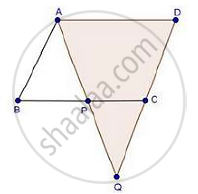Share
Notifications

View all notifications
Books Shortlist
Your shortlist is empty

# Solution for Abcd is a Parallelogram and Apq is a Straight Line Meeting Bc at P and Dc Produced at Q. Prove that the Rectangle Obtained by Bp and Dq is Equal to the Ab and Bc. - CBSE Class 10 - Mathematics

Login
Create free account

Forgot password?
ConceptCriteria for Similarity of Triangles

#### Question

ABCD is a parallelogram and APQ is a straight line meeting BC at P and DC produced at Q. Prove that the rectangle obtained by BP and DQ is equal to the AB and BC.

#### SolutionGiven: ABCD is a parallelogram

To prove: BP × DQ = AB × BC

Proof: In ΔABP and ΔQDA

∠B = ∠D                             [Opposite angles of parallelogram]

∠BAP = ∠AQD                     [Alternate interior angles]

Then, ΔABP ~ ΔQDA              [By AA similarity]

therefore"AB"/"QD"="BP"/"DA"                [Corresponding parts of similar Δ are proportional]

But, DA = BC                     [Opposite sides of parallelogram]

Then, therefore"AB"/"QD"="BP"/"BC"

⇒ AB × BC = QD × BP

Is there an error in this question or solution?

#### Video TutorialsVIEW ALL 

Solution Abcd is a Parallelogram and Apq is a Straight Line Meeting Bc at P and Dc Produced at Q. Prove that the Rectangle Obtained by Bp and Dq is Equal to the Ab and Bc. Concept: Criteria for Similarity of Triangles.
S